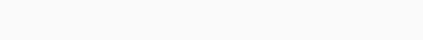# Solved – Group & Time Fixed Effects in a Difference-in-Difference Model

I'm fairly new to the field of econometrics and I am currently struggling to understand the literature regarding minimum wage policies and their effect on employment.

So the equation that I see in almost every paper looks like this:Now the part in their explanation I don't really understand are the county (group) and quarter (time) fixed effects. What are these fixed effects and what variable(s) from your dataset would you have to include to control for these fixed effects?

Contents

Welcome!

This specification is a more general adaptation of the difference-in-differences (DD) approach. If your data has a panel structure (i.e., multiple $$i$$ counties observed over $$t$$ quarters), then these variables would enter the model as dummies for $$each$$ county and $$each$$ quarter over the full panel series.

To be clear, $$phi_{i}$$ denotes unit (i.e., "county") fixed effects, while $$tau_{t}$$ denotes time (i.e., "quarter") fixed effects. County fixed effects accounts for unobserved, time-invariant county-level heterogeneity; time fixed effects accounts for common shocks, absent a minimum wage change, affecting all counties. Separate dummies for unit and time now give you county- and time-specific intercepts.

It is difficult to tell you exactly what variables to use without seeing your data, but I imagine you have the following variables (columns): county identifier, year, and quarter. Sometimes you might see a concatenated 'year-quarter' variable (e.g., 2018-Q1, 2018-Q2, … , etc.). Advising you on whether to use month, quarter, or year fixed effects is context specific. Nonetheless, separate indicators for county and time (i.e., quarters) is one way to estimate the equation you reference.

As for how to include these variables, it would depend upon the software you're using and how many $$i$$ counties and $$t$$ quarters the data contain. You could dummy code each county and time period manually if $$i$$ and $$t$$ are small. However, if the number of counties is large, you may want to let software do the work for you. If you're working in Stata, then you may want to leverage its time-series operators. If you're working in R, then you could use the following syntax:

``lm(y ~ x + as.factor(county) + as.factor(quarter) + covariates, data = ...) ``

where `as.factor()` 'dummies out' each county and time period for you, excluding one county and one time period to avoid collinearity.

I assume you are having trouble translating the equations into variables that you can work with in software. A paper by Wing and colleagues (2018) offers a concise review of different DD specifications (see pp. 456–457).

I hope this helps!

Rate this post